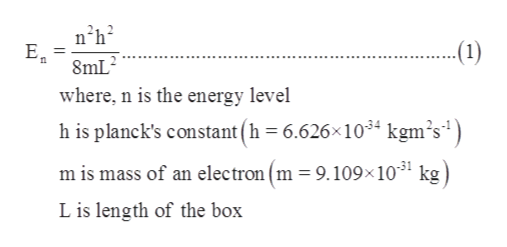# An electron in a one-dimenstional box requires a wavelength of 618 nm to excite an electron from the n=2 level to the n=4 level. Calculate the length of the box.

Question
49 views

An electron in a one-dimenstional box requires a wavelength of 618 nm to excite an electron from the n=2 level to the n=4 level. Calculate the length of the box.

check_circle

star
star
star
star
star
1 Rating
Step 1

It is given that:

Wavelength = 618 nm

Electron is exciting from n = 2 to n = 4 level

Step 2

The energy of an electron in one dimension is represented as follows:help_outlineImage Transcriptionclosen2h2 8mL where, n is the energy level h is planck's constant(h =6.626x1034 kgm's1 m is mass of an electron (m = 9.109x 1031 kg L is length of the box fullscreen
Step 3

Also, energy can be expressed in terms of Planck’s constant(...

### Want to see the full answer?

See Solution

#### Want to see this answer and more?

Solutions are written by subject experts who are available 24/7. Questions are typically answered within 1 hour.*

See Solution
*Response times may vary by subject and question.
Tagged in